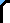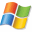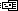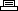Cheats DS GBA PC PS2 PS3 PSP Wii Xbox Xbox 360 New ReviewsSite Search

This Site Web
 Home: Game Cheats: PC GamesTurok: Dinosaur HunterEmail this Page  |Printer Friendly Version

```Entering Turok cheat codes is a snap! Simply goto the
main menu and enter code under Enter Cheat option.

Actions:                        Results:

D D D N S T B R           -->   INVINCIBILITY
N D N L P                 -->   SPIRIT MODE
T H M S C L S             -->   ALL WEAPONS
L N J H N S N             -->   UNLIMITED AMMO
R B C H N                 -->   BIG HEADS
G N N T R T M N T         -->   FULL MAP
J S N C R P N T R         -->   TINY ENEMY
C R L S F N D N G S       -->   PURDY COLORS
M T T S T B B N G T N B   -->   LEVEL 1 WARP
D R R N S T B B N G T N   -->   LEVEL 2 WARP
M K J N K                 -->   LEVEL 3 WARP
P T N D N C N             -->   LEVEL 4 WARP
N N T N D                 -->   LEVEL 5 WARP
T R M N N                 -->   LEVEL 6 WARP
J M D N N                 -->   LEVEL 7 WARP
M R G R T T R R           -->   LEVEL 8 WARP
S H N T R R N T           -->   GALLERY
T H T R T M B B           -->   SHOW CREDITS
J F F S P N G D N B R G   -->   INFINITE LIVES
D N L D D C K             -->   QUACK MODE
N G L C K C K             -->   ALL KEYS
J H N T H M S             -->   LONGHUNTER WARP
S T P H N B R M L         -->   MANTIS WARP
G R G M L C H C K         -->   TREX WARP
R N T R C                 -->   CAMPAIGNER WARP
B K S T R D               -->   FLY MODE
F H G T B C               -->   SHOW ENEMIES
J H N N C K               -->   DISCO MODE```